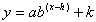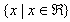Introduction

As discussed previously, for exponential functions of the form,, there is no restriction on the value of x. Therefore, the function is defined for all real values of x, and the domain is.

All exponential functions have an asymptote, or line that the graph will approach but never touch or cross. Therefore, the range of an exponential function of the form,, is dependent on the sign of a and the value of the asymptote. If a is a positive value then the graph will approach the asymptote from the top, which means the range will be greater than the asymptote. If a is negative, then the graph will approach the asymptote from the bottom, which means the range will be less than the asymptote. Because the graph never intersects the asymptote, the range will never include this value.

Listen